Introduction to Java

Environments

Chapter 1

This HTML is created from PDF at https://www.pdfonline.com/convert-pdf-to-html/

Java Environments

1)Java API - Application Programming Interface

provides the core functionality of the Java programming language.

contains predefined classes

it is group into libraries - called package.

2)JDK - Java Development Kit

A software that contains development tools for develop and execute java program (Java Runtime Environment (JRE) + Java Virtual Machine (JVM)+ library classes)

This HTML is created from PDF at https://www.pdfonline.com/convert-pdf-to-html/

Java Environments (con’t)

3)Java IDE - Integrated Development Environment

Known as editor too - to write program

Many IDEs in the market already bundled with JDK

NetBeans: JDK 8u111 with NetBeans 8.2

This HTML is created from PDF at https://www.pdfonline.com/convert-pdf-to-html/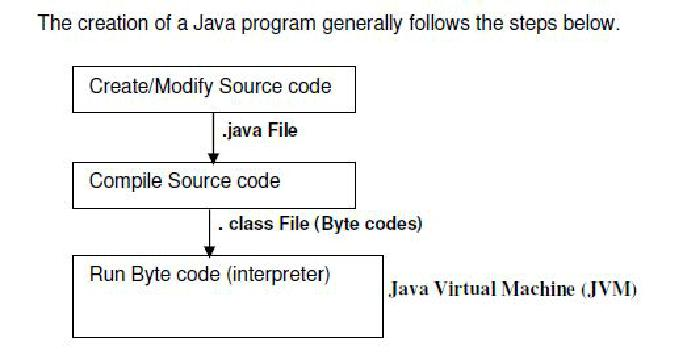Creating, Compiling and Executing

This HTML is created from PDF at https://www.pdfonline.com/convert-pdf-to-html/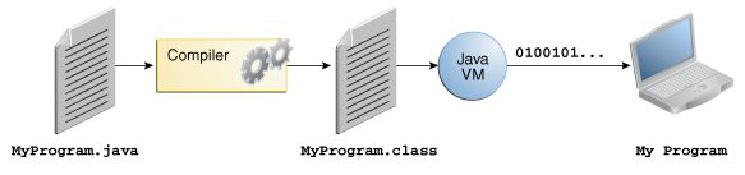Creating, Compiling and Executing

This HTML is created from PDF at https://www.pdfonline.com/convert-pdf-to-html/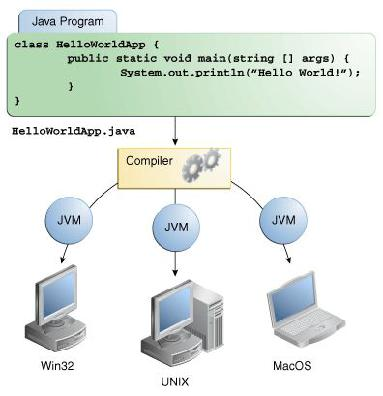Creating, Compiling and Executing

Through the JVM, the same application is capable of running on multiple platforms >> platform independent language

This HTML is created from PDF at https://www.pdfonline.com/convert-pdf-to-html/

Basic Input & Output

This HTML is created from PDF at https://www.pdfonline.com/convert-pdf-to-html/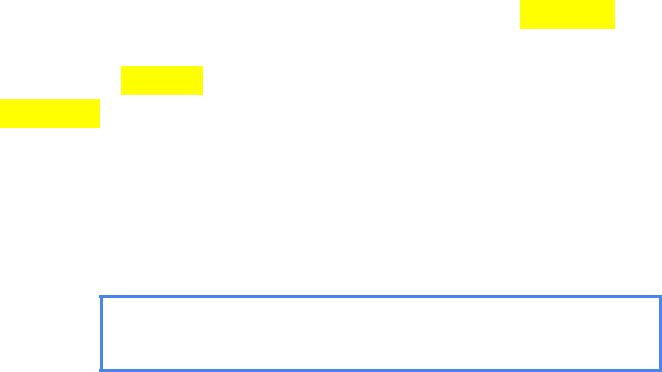Getting input from user (keyboard)

There are various way to read input from keyboard. One of them is Scanner class.

Scanner is found in package java.util

Scanner provides methods to read and parse various primitive values (int, double, float, etc).

nextInt()

nextDouble()

nextFloat()

nextBoolean()

next()

nextLine()

This HTML is created from PDF at https://www.pdfonline.com/convert-pdf-to-html/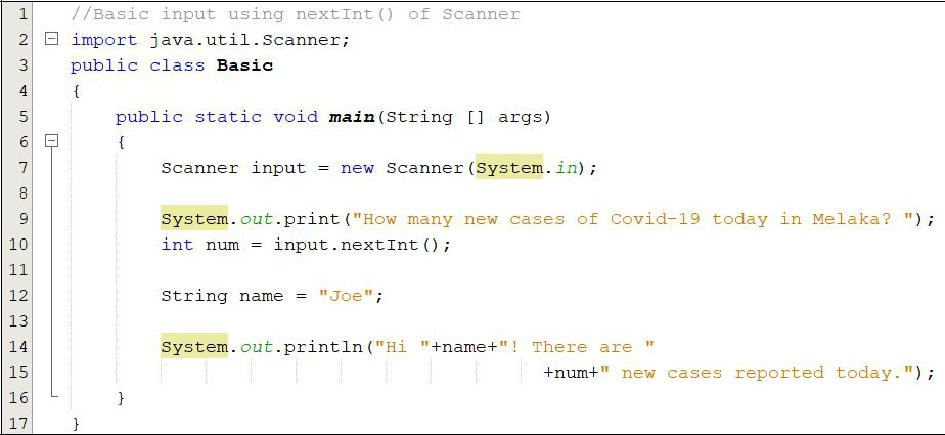How to apply?

This HTML is created from PDF at https://www.pdfonline.com/convert-pdf-to-html/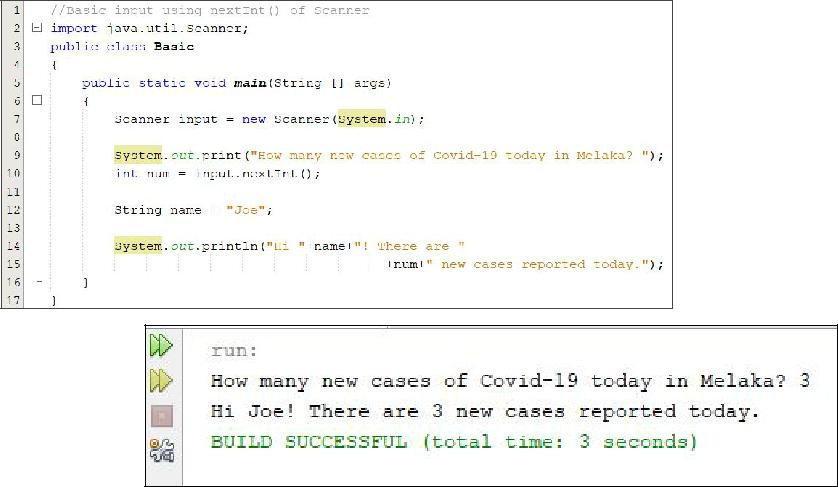Output

This HTML is created from PDF at https://www.pdfonline.com/convert-pdf-to-html/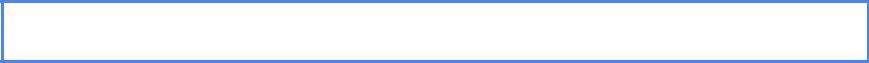Line 7: Scanner input = new Scanner(System.in);

“input” is an instance (object) of Scanner class.

We need the object to access (use) the methods in Scanner class.

Without it, you can’t access the methods.

This HTML is created from PDF at https://www.pdfonline.com/convert-pdf-to-html/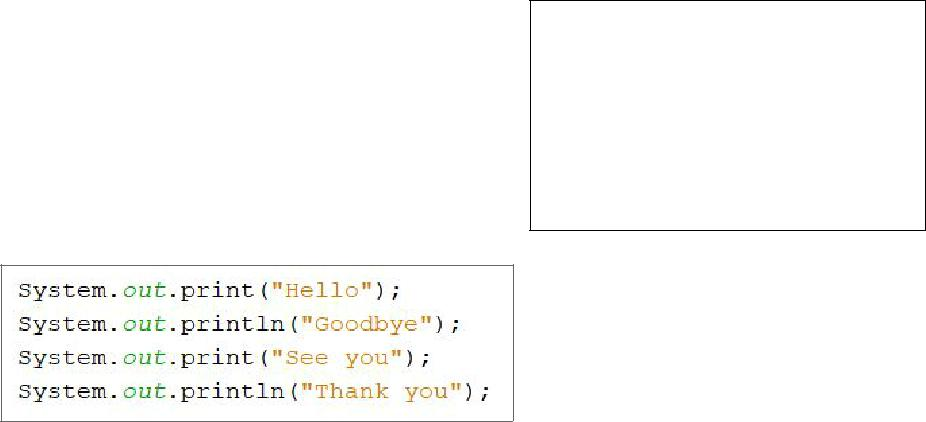Output - To display to screen

Example 1

Methods : System.out. :

print();

println();

printf();

Output?

This HTML is created from PDF at https://www.pdfonline.com/convert-pdf-to-html/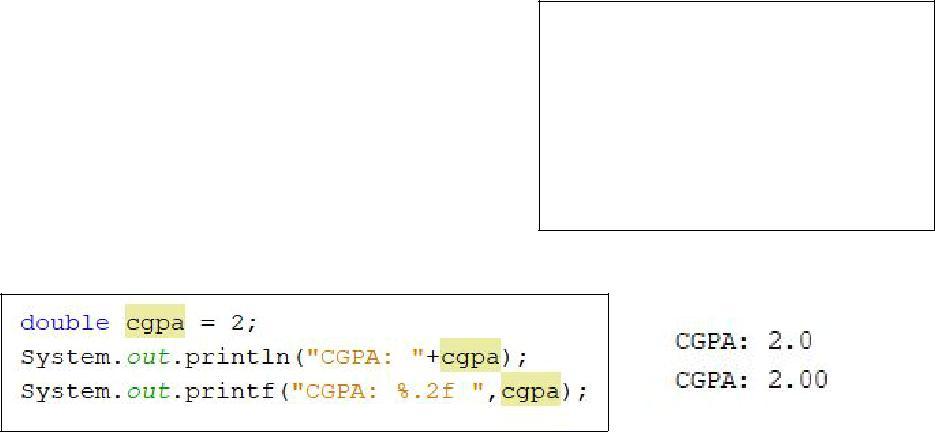Output - To display to screen (con’t)

Example 2

Methods : System.out. :

print();

println();

printf();

Output?

This HTML is created from PDF at https://www.pdfonline.com/convert-pdf-to-html/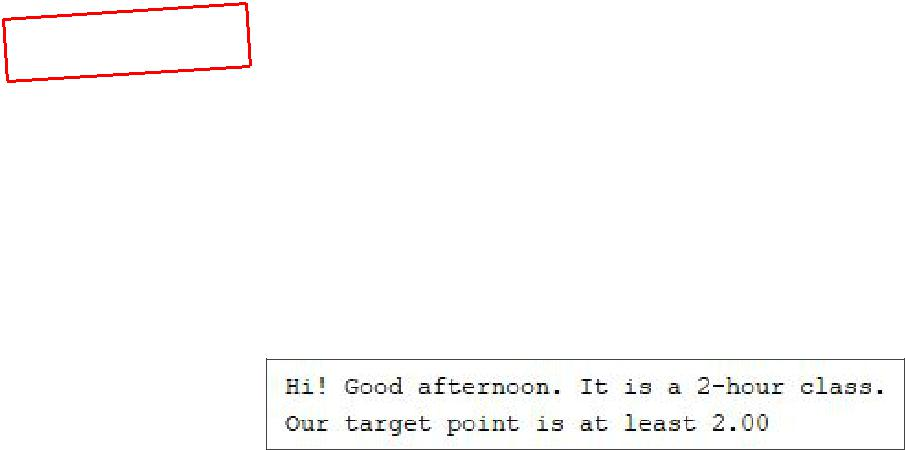2.Based on the following declaration, write the code to display an output as shown below. Use only two lines of code.

String greeting = "afternoon"; int hour = 2;

double target = 2;

This HTML is created from PDF at https://www.pdfonline.com/convert-pdf-to-html/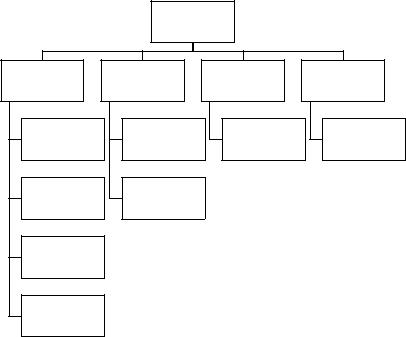DPJ 5018 – Programming in Java Chapter 2

Chapter 2: REVISION OF FUNDAMENTAL IN PROGRAMMING (Data, Operations and Control Structures)

Identifiers

You need to choose names for the things you will refer to in your programs. Identifiers are used for naming programming entities such as variables, constants, methods, classes, and packages. Here are the rules for naming identifiers:

Can be a sequence of characters that consist of letters, digits, underscores (_) and a dollar sign (\$).

Must start with a letter, an underscore or dollar sign. It cannot begin with a digit.

Cannot be a reserved word. (Java language keywords : http://docs.oracle.com/javase/tutorial/java/nutsandbolts/_keywords.html)

Cannot be ‘true’, ‘false’ or null.

Can be of any length.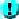Note: Since java is case-sensitive, X and x are different identifiers.

Data Types

The Java language provides eight primitive types of data:

Data Types

Integer

Floating

Character

Boolean

byte

float

char

boolean

short

double

int

long

 CDP Page 1 of 17 as/5018/Tri61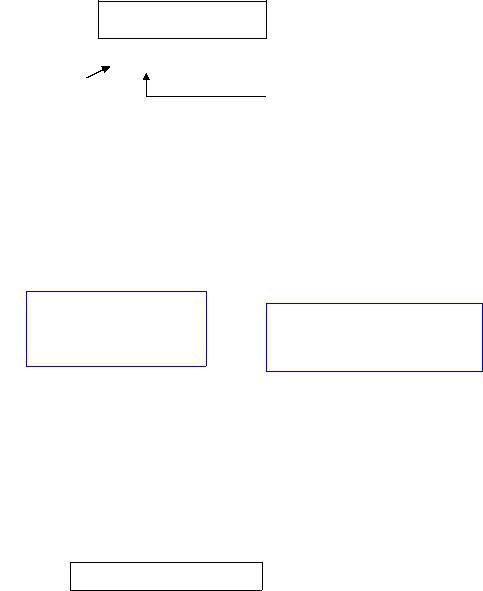DPJ 5018 – Programming in Java Chapter 2

Variables

Data is variable when its value may change during program execution. They are used to store data input, output or intermediate data.

Declaring variables

To use a variable, you declare it by telling the compiler the name of the variable as well as the type of data it represents.

The computer will allocate appropriate memory space for the variable based on its data type.

HOW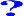Syntax:

datatype variableName;

Example:

int age;

integerFollow the rules for naming a variable

Other examples:

double oldPrice; double radius; int qty;

Short-hand form:

If variables are the same type, they can be declared together (separated by commas), as follows:

int myAge;

int ageGrandpa ; double bookPrice; double pricePayed;

= =

int myAge, ageGrandpa; double bookPrice, pricePayed;Note: 1) In Java naming convention, variable names are in lowercase. 2) A variable must be declared before it can be assigned a value.

Assignment Statements and Expressions

After a variable is declared, you can assign a value to it by using an assignment statement (=). Refer to the syntax below:

variableName = expression;

 CDP Page 2 of 17 as/5018/Tri61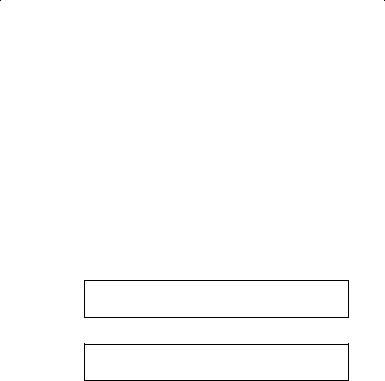DPJ 5018 – Programming in Java Chapter 2

Expression – represents a computation involving values, variables, and operators that evaluates to a value. For example:

 int x = 5; //assign 5 to a variable x double radius = 2.3; //assign 2.3 to a variable radius char a = ‘A’; //assign ‘A’ to a variable a x = 5 * (2/3) + 5; //assign the value of the expression to x area = radius * radius * 3.142; //compute area

Constants

The value of a variable may change during program execution, but a constant represents permanent data that will never be changed. For example, to compute area of a circle - π is a constant.

If you don’t want to keep typing 3.142; instead you can define a constant for π.

HOWSyntax:

final datatype constantName = VALUE;

Example:

final double PI = 3.142;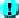Note: 1) The word final is a Java Keyword, which means that the constant cannot be changed.

2)In Java naming convention, constants are named in uppercase: PI

A constant must be declared and initialized before it can be used.

Benefits of using constants:

oYou don’t have to repeatedly type the same value

oThe value can be changed in a single location, if necessary o The program is easy to read.

 CDP Page 3 of 17 as/5018/Tri61

This HTML is created from PDF at https://www.pdfonline.com/convert-pdf-to-html/DPJ 5018 – Programming in Java Chapter 2

Declare and display value

//DemoVariables.java

//Declare and display value

public class DemoVariables

{

public static void main(String[] args)

{

int d = 315; System.out.println(“The int is”); System.out.println(d);

//or u can write like this

// System.out.println(“The integer is” + d);

}

}

Declare two more variables in a program

//DemoVariables2.java

//Declare and display value

public class DemoVariables2

{

public static void main(String[] args)

{

int oneInt = 315; short oneShort = 23;

long oneLong = 1234567876543L;

System.out.println(“The int is ”);

System.out.println(oneInt);

System.out.println(“The short is” + oneShort);

System.out.println(“The long is ”);

System.out.println(oneLong);

}

}Note:

The plus (+) in the System.out.println(“ ”) statement means to concatenate strings if one of the operands is a string. If both operands are numbers, the + operator will add them.

 CDP Page 4 of 17 as/5018/Tri61

This HTML is created from PDF at https://www.pdfonline.com/convert-pdf-to-html/DPJ 5018 – Programming in Java Chapter 2

Arithmetic Statements

There are five standard arithmetic operators in Java: o addition (+)

o subtraction (-) o division (/)

o multiplication(*)

o modulus (%). The modulus operator is for integers, and returns the remainder of integer division

 Operator Description Example + Addition 45 + 5, result: 50 - Subtraction 100 – 80, result: 20 * Multiplication 8 * 5, result: 40 / Division 43/2, result: 21 (not 21.5) % Modulus (remainder) 43%2, result: 1 (cause remainder is 1)

The common shortcut operators:

 Operator Description Example Equivalent += Addition assignment i +=7 i = i+7 -= Subtraction “ f -=3.5 f = f-3.5 *= Multiplication “ k *=5 k = k*5 /= Division “ s /=4 s = s/4 %= Remainder “ n %=2 n = n%2 x++ Suffix (incrementing) x=x+1 ++x Prefix x=x+1

** same as (x- -) and (- -x)

 CDP Page 5 of 17 as/5018/Tri61

This HTML is created from PDF at https://www.pdfonline.com/convert-pdf-to-html/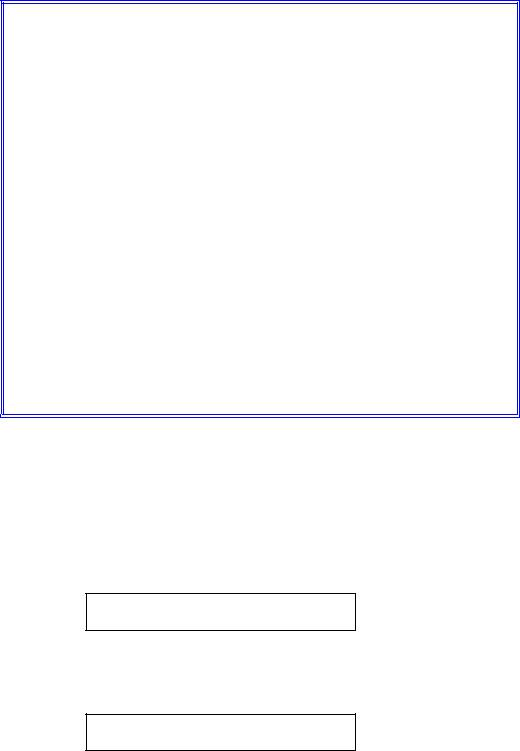DPJ 5018 – Programming in Java Chapter 2

Example:

//DemoVariables3.java

//use arithmetic statement

public class DemoVariables3

{

public static void main(String[] args)

{

int value1 = 43, value2 = 10, sum, difference, product, quotient, modulus;

sum = value1 + value2; difference = value1 – value2; product = value1 * value2; quotient = value1 / value2; modulus = value1 % value2;

System.out.println(“Sum is ” + sum);

System.out.println(“Difference is ” + difference);

System.out.println(“Product is ” + product);

System.out.println(“Quotient is ” + quotient);

System.out.println(“Modulus is ” + modulus);

}

}

When mathematical operators are combined in an expression, you must understand the effects of operator precedence. In the absence of parenthesis, multiplication, division and modulus operations will be done before addition and subtraction operations.

The Boolean data type

A Boolean variable may contain only one of two values - true or false.

boolean isInOffice = false; boolean isOutOffice = true;

Another example, the following statement displays true.

System.out.println (1<2);

 CDP Page 6 of 17 as/5018/Tri61

This HTML is created from PDF at https://www.pdfonline.com/convert-pdf-to-html/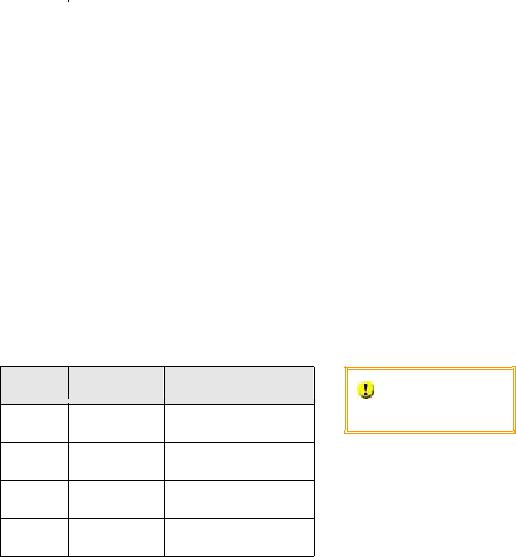DPJ 5018 – Programming in Java Chapter 2

Java supports six comparison operators to be used in comparing two data items, equal to (==), greater than (>), less than (<), not equal (!=), greater or equal (>=), less than or equal (<=). Expressions which contain comparison operators result in a Boolean value, refer to the table below.Note:

The equality comparison operator is two equal signs (==), not a single equal sign

(=). The latter symbol is for assignment.

Logical operators:

 Operator Description true Example false Example < Less than 2<10 10>2 > Greater than 10>2 2>10 == Equal to 1 == 1 1 == 2 Less than or equal <= to 5<=5 9<=1 Greater than or >= equal to 5>=2 2>=5 != Not equal to 4 != 3 1 != 1

Boolean Operators:

 Operator Name Description Do a Truth Table for each Operator. ! not Logical negation && and Logical conjunction || or Logical disjunction ^ exclusive or Logical exclusion
 CDP Page 7 of 17 as/5018/Tri61

This HTML is created from PDF at https://www.pdfonline.com/convert-pdf-to-html/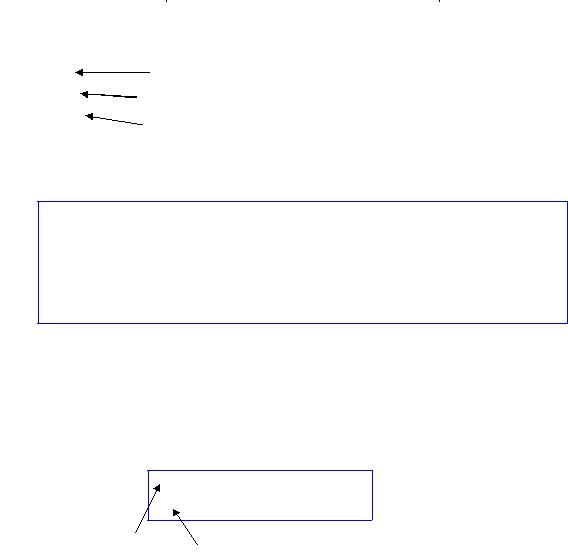DPJ 5018 – Programming in Java Chapter 2

Floating point data types

Floating-point data types are used to store values that contain decimal positions. Java supports two floating-point types, float and double.

A float data type can represent 6 or 7 significant digits of precision, while a double can represent 14 or 15 significant digits of precision.

 Type Minimum Maximum Size in bytes Float -3.4 * 1038 3.4 * 1038 4 Double -1.7 * 10308 1.7 * 10308 8 Default: int 178 21.8 Default: double 21.8F float

Example:

double dnum1 = 1.5, dnum2 = 0.5, dResult;

dResult = dnum1 + dnum2;

System.out.println(“the sum of the doubles is ” + dResult); dResult = dnum1 * dnum2;

System.out.println(“the product of the doubles is ” + dResult);Modulus operations should not be performed on floating point data

Numeric type conversion

When performing arithmetic on variables and constants of the same type, the result of the operation retains that type. Example:

Result1 = 10 / 5

Result2 = 15.8 – 10.5

float

int

When performing operations on operands of unlike types, Java selects a unifying type for the result. Java then implicitly, or automatically, converts the nonconforming operands to the unifying type.

 CDP Page 8 of 17 as/5018/Tri61

This HTML is created from PDF at https://www.pdfonline.com/convert-pdf-to-html/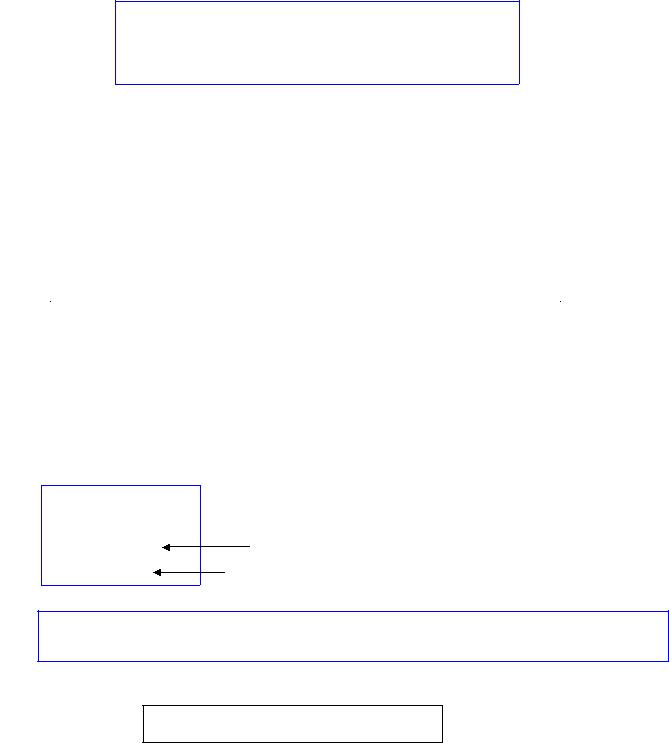DPJ 5018 – Programming in Java Chapter 2

int quantity = 5; double price = 2.00;

double totalPrice = quantity * price;

List of data type in order for establishing unifying types between two variables.

1.double

2.float

3.long

4.int

5.short

6.byte

You can explicitly override the unifying type by placing the desired result type in parenthesis before the variable or constant to cast. This is called type casting.

 double income = 127.00; float share = (float) income / 5; // 25.40 int ringgitPerPerson = (int) share; //ringgitPerPerson is 25

The char data type

Char: character

Use the char data type to store a single character.

char aChar = ‘9’; int aNum = 9;

 char aChar = 9; Output: a blank int aNum = ‘9’; Output: 57 (ASCII code)

char myInitial = ‘A’; char perssign = ‘%’ ; char numChar = ‘9’ ;

The char type is only for single characters. To store a string, you must use the String data structure.

String myName = “Fatima”;Note: A string must be enclosed in quotation marks. So, “A” is a string and ‘A’ is a character.

 CDP Page 9 of 17 as/5018/Tri61

This HTML is created from PDF at https://www.pdfonline.com/convert-pdf-to-html/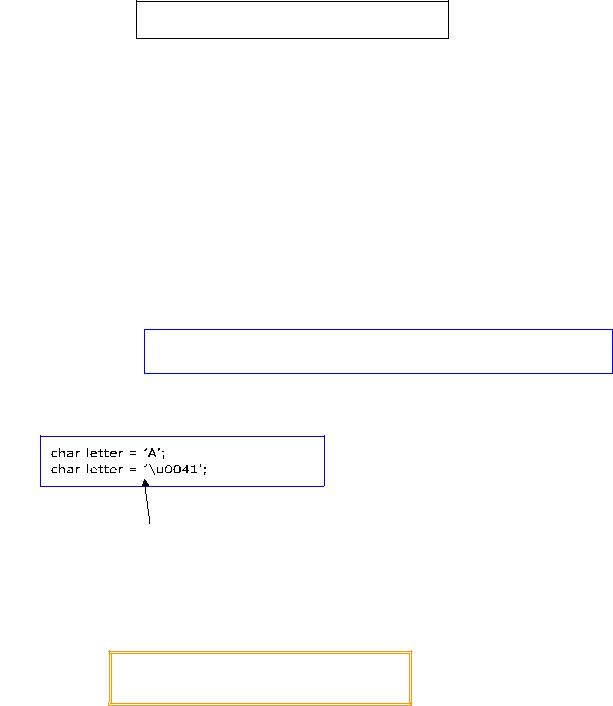DPJ 5018 – Programming in Java Chapter 2

Characters may be assigned to char variables, or assignments may be made using an escape sequence containing the Unicode value of the character. Non-standard and international characters may be assigned in this way.

char aBackSpaceChar = ‘\b’ ;

Escape sequences are to represent special characters as shown in the table below. It has a special meaning to the compiler.

 Escape Sequence Description \b Backspace \t Tab \n Newline or linefeed \f Form feed \r Carriage return \” Double quotation mark \’ Single quotation mark \\ Backslash

For example, you want to print the quoted message:

They shouted “Malaysia Boleh”

• The statement: System.out.println(“They shouted \”Malaysia Boleh\””);

The characters used in Java are represented in Unicode, which is a 16-bit coding scheme for characters.

Same: variable letter stores character A

Escape sequence: \u

Operator Precedence and Associativity

Determine the order in which operators are evaluated.

• For example: 3 + 4 * 4 > 5 * ( 4 + 3 ) – 1 What’s the answer?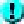Find a table/chart of Operator Precedence

 CDP Page 10 of 17 as/5018/Tri61

This HTML is created from PDF at https://www.pdfonline.com/convert-pdf-to-html/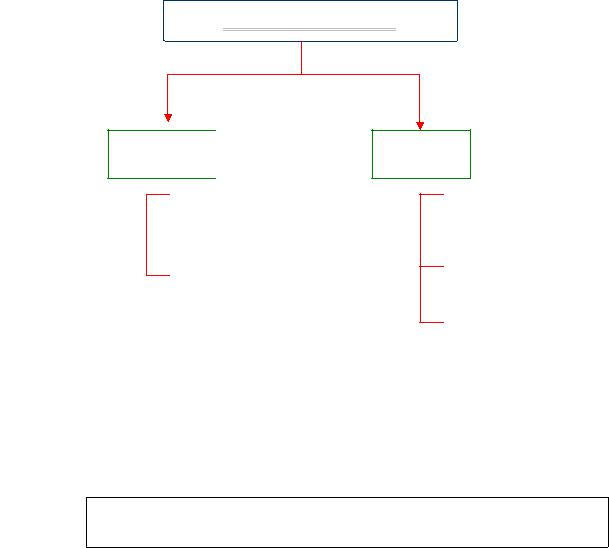DPJ 5018 – Programming in Java Chapter 2

CONTROL STRUCTURES

Introduction

The order in which statements are executed in a running program is called the flow of control. Unless otherwise specified, the execution of a program proceeds in a linear fashion. We can alter the flow of control through the code by using certain types of programming statements. Java has 2 categories of control structures:

Selection: let you choose actions with two or more alternative courses.

Repetition/Loop: control the repeated execution of statements.

In this chapter, you will learn various selection and loop control statements.

Control Structures

Selection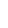if - else

switch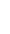Selection Statements

Loopwhile

do - while

for

Java has several types of selection statements: simple if statements, if…else statements, nested if statements, switch statements and conditional statements.

Simple if statements

Simple if statement executes an action only if the condition is true.

The syntax:

if (booleanExpression) { statement(s);

}

 CDP Page 11 of 17 as/5018/Tri61

This HTML is created from PDF at https://www.pdfonline.com/convert-pdf-to-html/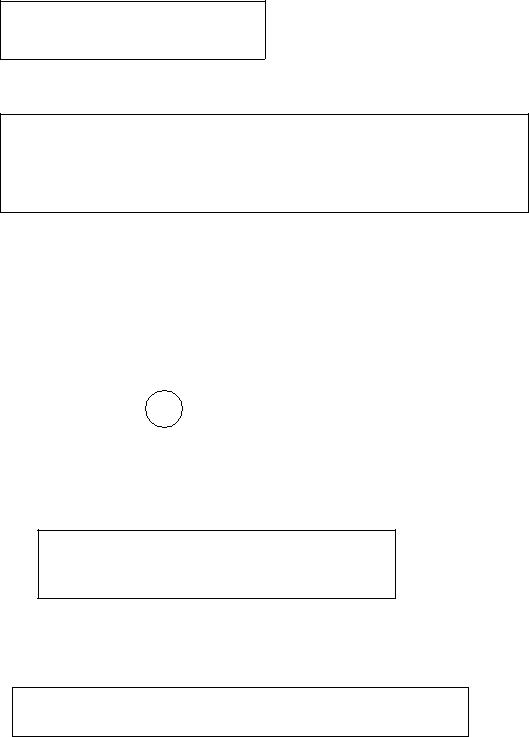DPJ 5018 – Programming in Java Chapter 2

Simple example:

System.out.println(“Passed”);

Another Example:

area = radius * radius* PI; System.out.println (“The area is “ + area);

}

System.out.println (“Thank you”);Note: 1) If the value of radius is greater than or equal to 0, then the area is computed the result and ‘Thank you’ are displayed.

2)Otherwise, the two statements in the block will not be executed. Only the

‘Thank you’ is displayed.

CAUTION:

1)Adding a semicolon at the end of an if clause is a common mistake. For example,

 If (grade <=80) ; logic error

oThis mistake is hard to find, because it is not a compilation error or runtime error, it is logic error.

2)The example below does not have syntax errors. It assigns true to e so that e is always true.

if ( e = true)

System.out.println (“ It is OK ”);Note:

1)If we include the Boolean expression, we need to enclose in parentheses for all forms of the if statement. For example:

if ( ( i>0) && ( i<0) )

System.out.println (“ i is an integer between 0 and 10 ”);

2) The braces { } can be omitted if there is only ONE statement within them.

 CDP Page 12 of 17 as/5018/Tri61

This HTML is created from PDF at https://www.pdfonline.com/convert-pdf-to-html/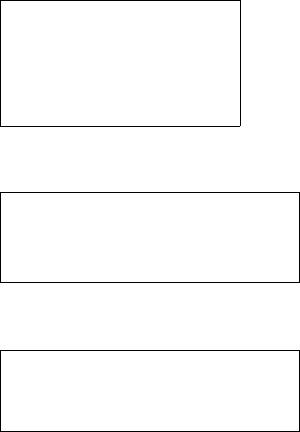DPJ 5018 – Programming in Java Chapter 2

If…else statements

A simple if statement takes an action if the specified condition is true. If the condition is false, nothing is done. But if you want to take alternative actions when condition is false? You can use an if …else statement

The actions that an if…else statement specifies differ based on whether the condition is true or false.

The syntax:

if (booleanExpression) { statement(s) for the true case;

}

else {

statement(s) for the false case;

}

Example:

else System.out.println(“Failed”);

Another Example:

if (number % 2 == 0)

System.out.println ( number + “ is even.”); else

System.out.println ( number + “ is odd.”);

If number % 2 is 0, the message number is even is printed, if it is false, the odd number is printed.HOW TO COMPARE BETWEEN STRINGS?

Nested if statements

The statement can be used to implement multiple alternatives.

Nested if can contain another if statement, in fact there is no limit to the depth of the nesting.

 CDP Page 13 of 17 as/5018/Tri61

This HTML is created from PDF at https://www.pdfonline.com/convert-pdf-to-html/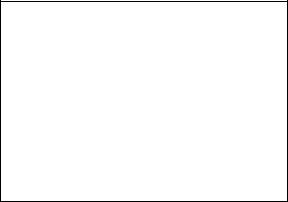DPJ 5018 – Programming in Java Chapter 2

Example:

else if (score >= 80.0) grade = ‘B’;

else if (score >= 70.0) grade = ‘C’;

else if (score >= 60.0) grade = ‘D’;

elseNote:

1)As usual, the braces can be omitted if there is only one statement within them.

2)This is the preferred writing style for multiple alternative if statements. It avoids deep indentation and makes the program easy to read.

 CDP Page 14 of 17 as/5018/Tri61

This HTML is created from PDF at https://www.pdfonline.com/convert-pdf-to-html/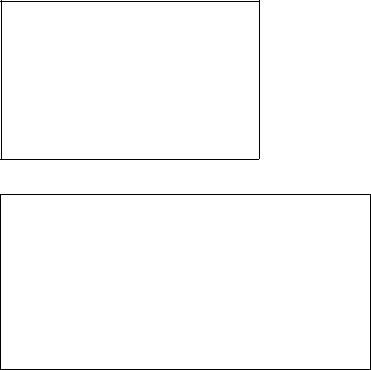DPJ 5018 – Programming in Java Chapter 2

Switch statements

Overuse of nested if statement makes a program difficult to read. Java provides a switch statement to handle multiple conditions efficiently.

The syntax:

switch ( switch-expression)

{case value1 : statement(s)1; break;

case value2 : statement(s)2; break;

case valueN : statement(s)N; break;

……

default : statement(s)-for default;

}

Example:

switch (years)

{ case 5 : bonusrate = 0.25; break;

case 7 : bonusrate = 0.50; break;

case 8: bonusrate = 0.75; break;

……

default : System.out.println(”Wrong number of years”);

}

The switch statement observes the following rules:

oThe switch–expression must yield a value of char, byte, short, or int type and must always be enclosed in parentheses ( ).

oThe value1, value2…..valueN must have the same data type as the value of the switch-expression.

oThe keyword break is optional, but it should be used at the end of each case in order to terminate the remainder of the switch statement. If the break

statement is not present, the next case statement will be executed.

oThe default case, which is optional, can be used to perform actions when none of the specified cases matches the switch-expression.

oThe order of the cases (including the default case) does not matter. However, it is good programming style to follow the logical sequence of the cases and place the default case at the end.

CAUTION:

oDo not forget to use a break statement when one is needed. If it is not present, the next case statement will be executed.

 CDP Page 15 of 17 as/5018/Tri61

This HTML is created from PDF at https://www.pdfonline.com/convert-pdf-to-html/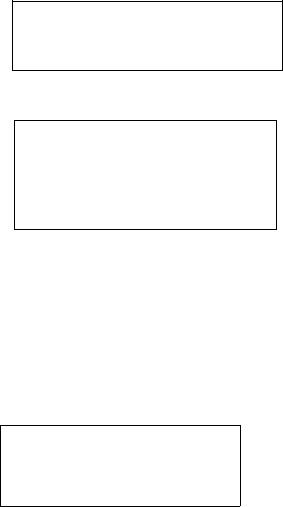DPJ 5018 – Programming in Java Chapter 2

Loop Statements

Loops are structures that control repeated executions of a block of statements. The part of the loop that contains the statements to be repeated is called the loop body.

Each loop contains a Boolean expression (loop-continuation-condition) that controls the execution of the body.

If the condition is true, the execution of the body is repeated. If false, the loop terminates.

Java provides three types of loop statements: the while loop, the do-while loop and the for loop.

The while loop

The syntax:

while (loop-continuation-condition) { statement(s);//loop body

}

Example:

int i = 0; do {

System.out.println(“Good day!”); i++;

} while ( i<5);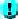NOTE:

oThe do-while loop executes the loop body first, and then checks the loop condition to determine whether to continue or terminate the loop.

The for loop

The syntax:

for (i= initialValue; i <endValue; i++)

{

statement(s); //loop body

}

 CDP Page 16 of 17 as/5018/Tri61

This HTML is created from PDF at https://www.pdfonline.com/convert-pdf-to-html/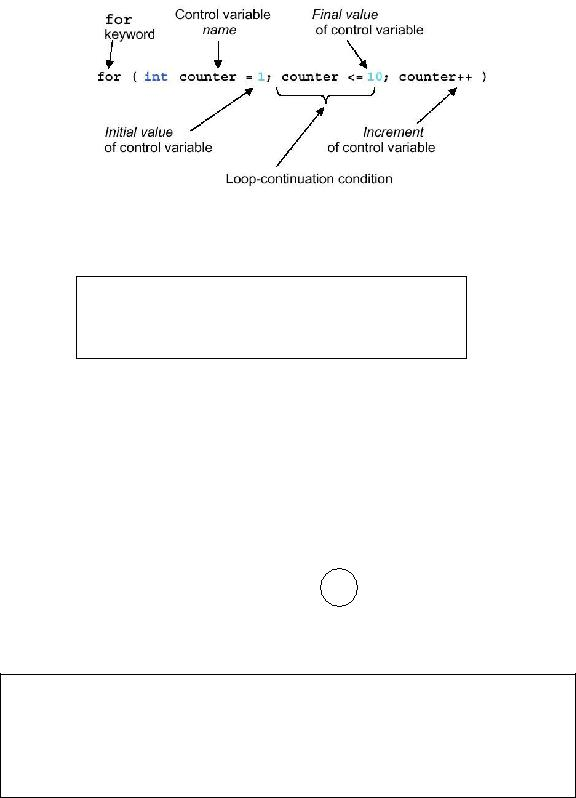DPJ 5018 – Programming in Java Chapter 2

Flowchart: (same as while loop)

Example:

int i;

for ( i = 0; i<5; i++) {

System.out.println(“Good day!”);

}NOTE:

1)The for loop statement starts with the keyword for, followed a pair of parentheses.

2)Each statement is separated by semicolons.

3)The flow is same with while loop.

CAUTION:

o Adding a semicolon at the end of the for clause before the loop body is a common mistake.

for (int n = 0; n<10; n++) ;

References:

1.Y.Daniel Liang. Introduction to JAVA Programming, 8th Edition (International Edition), Prentice Hall, 2010.

2.(Harvey & Paul) M Deitel & Deitel, Java™ How To Program: Early Objects Version, 8th Edition, Prentice Hall, 2009.

3.Ralph Morelli and Ralph Walde, Java, Java, Java, Object-Oriented Problem Solving, 3rd Edition, Prentice Hall, 2005.

4.Bruce Eckel, Thinking in Java, 4th Edition, Prentice-Hall, 2006.

 CDP Page 17 of 17 as/5018/Tri61

This HTML is created from PDF at https://www.pdfonline.com/convert-pdf-to-html/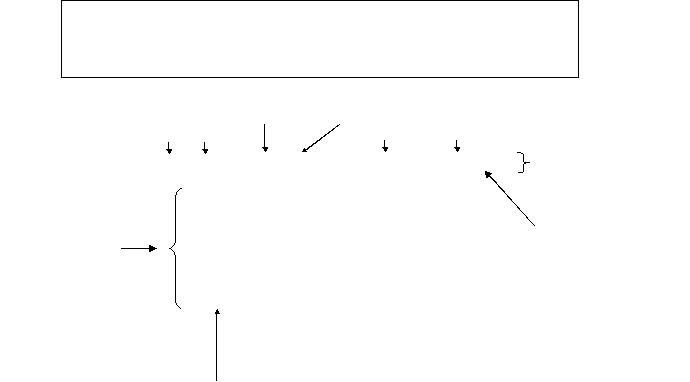DPJ 5018 – Programming in Java Chapter 3 Part 1

Chapter 3: METHODS and ARRAYS

METHODS

Introduction

A method is a collection of statements that are grouped together to perform an operation. For example, when you call the System.out.println() method, the system actually executes several statements in order to display a message on the console.

Creating a Method

Syntax / structure:

modifier returnValuetype methodname (list of parameters)

{

//method body

}

 • Example: return value type method name Parameters public static int max (int num1, int num2) method { modifier header int result; if ( num1>num2 ) method result = num1; signature method body else result = num2; return result; }

return value

Modifier

Optional, tells the compiler how to call the method.

static modifier is used (will be introduced in Chapter 4).

Return value type

The data type of the value the method returns.

If the method does not return a value, the return value type is the keyword void.

All methods except constructors requires return value type. Constructors will be introduced in Chapter 4.

 CDP Page 1 of 15 ainee/5018/Tri61

This HTML is created from PDF at https://www.pdfonline.com/convert-pdf-to-html/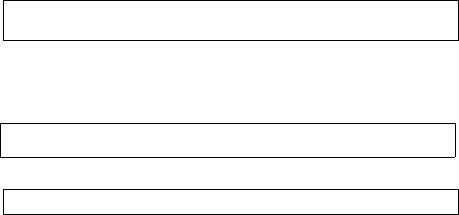DPJ 5018 – Programming in Java Chapter 3 Part 1

Parameters

Refers to the type, order and number of the parameters of a method.

Parameters are optional; A method may contain no parameters.

When a method is invoked, you pass a value to the parameter. This value is referred to as actual parameter or argument.

Method body

Contains a collection of statements that define what the method does.

A return statement using the keyword return is required for a non-void method to return a result.

The method terminates when a return statement is executed.CAUTION:

You need to declare a separate data type for each parameter. For instance, int num1, num2 should be replaced by int num1, int num2.

Note: In certain other languages, methods are referred to as procedures or functions.

Calling a Method

To use a method, you have to call or invoke it. Two ways to call a method:

If the method returns a value

int larger = max(3,4); double m = convertMeter(km);

//it calls convertMeter( ) and assigns the result to the variable m

OR

System.out.println (max(3,4));

System.out.println (convertMeter(km));

If the method returns void, a call to the method must be a statement. Example: convertMeter(km);

When a program calls a method, program control is transferred to the called method. A called method returns control to the caller when its return statement is executed or when its method- ending closing brace is reached.

Refer to the example below: To test the max method:

 CDP Page 2 of 15 ainee/5018/Tri61

This HTML is created from PDF at https://www.pdfonline.com/convert-pdf-to-html/DPJ 5018 – Programming in Java Chapter 3 Part 1

Problem:

Write a program that demonstrates how to create and invoke the max method. The method will return the maximum number.

Solution: (Source – Text book page 128)

//Chapter 2 eg1: TestMax.java

public class TestMax {

public static void main (String [] arg)

{

int i = 5; int j = 2;

int k = max(i, j);

System.out.println(“The maximum between ” +i+ “ and ” +j+ “ is ” +k); } //close main

public static int max (int num1, int num2)

{

int result;

if ( num1>num2 ) result = num1;

else

result =num2; return result;

}

} //close class

Explanation:

This program contains the main method and max method.

In this example, the main method invokes max (i, j) which is defined in the same class with the main method.

When the max method is invoked, i and j values are passed to num1 and num2. The flow of control transfers to the max method.

The max is executed. When the return statement is executed, it returns the control to its caller (main) and the value of result is passed to variable k.

Passing Parameters

The power of a method is its ability to work with parameters. When invoking a method with parameters, a copy of the value of the actual parameter is passed to the method. This is referred to as pass by value. The actual variable outside the method is not affected, regardless of the changes made to the parameter inside the method.

 CDP Page 3 of 15 ainee/5018/Tri61

This HTML is created from PDF at https://www.pdfonline.com/convert-pdf-to-html/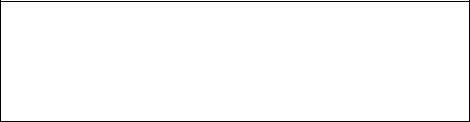DPJ 5018 – Programming in Java Chapter 3 Part 1

Analyze the program:(TestMax.java)

The max method works only with the int data type. But if we need to find which of two floating-point numbers has the maximum value? The solution is to create (add) another method with the same name but different parameters in the program.

public static double max (double num1, double num2)

{

if(num1> num2) return num1;

else

return num2;

}

If you call max with the double parameters, the max method that expects double parameters will be invoked. This is referred to as method overloading; that is two methods have the same name but different parameters. Refer to the example below:

 CDP Page 4 of 15 ainee/5018/Tri61

This HTML is created from PDF at https://www.pdfonline.com/convert-pdf-to-html/DPJ 5018 – Programming in Java Chapter 3 Part 1

public static void main(String[] args) {

//Invoke the max method with int parameters System.out.println("The maximum between 3 and 4 is " + max(3, 4));

//Invoke the max method with double parameters

System.out.println("The maximum between 3.0 and 5.4 is "+ max(3.0, 5.4));

// Invoke the max method with three double parameters System.out.println("The maximum between 3.0, 5. 4, and 10.14 is "+ max(3.0,

5.4, 10.14));

}

/** Return the max between two int values */

public static int max(int num1, int num2) { if (num1 > num2)

return num1; else

return num2;

}

/** Find the max between two double values */

public static double max(double num1, double num2) { if (num1 > num2)

return num1; else

return num2;

}

/** Return the max among three double values */

public static double max(double num1, double num2, double num3) { return max(max(num1, num2), num3);

}

}Note

Overloaded methods must have different parameter lists. You cannot overload methods based on different modifiers or return types.

 CDP Page 5 of 15 ainee/5018/Tri61

This HTML is created from PDF at https://www.pdfonline.com/convert-pdf-to-html/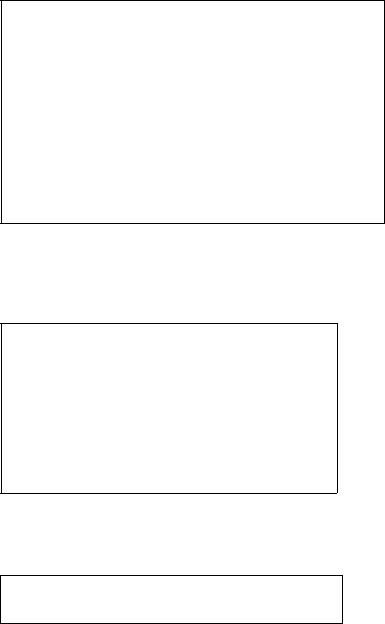DPJ 5018 – Programming in Java Chapter 3 Part 1

The scope of local variables

oThe scope of variable is the portion of the program where the variable can be accessed.

oIs limited locally to a method. It starts from its declaration and continues to the end of the block that contains the variable.

oA local variable must be declared before it can be used. o It must be initialized before it is referenced.

o You will learn about instance variables and class variables in Chapter 3.

You can declare a local variable with the same name multiple times in different non-nesting blocks in a method, but you cannot declare a local variable twice in nested blocks. Thus, the following code is correct.

public static void correctMethod( )

{

int x=1; int y=1;

// i is declared

for (int i = 1; i<10; i++) x += i;

//i is declared again for (int i = 1; i<10; i++)

y += i;

}

The next code would cause a compilation error because variable i is declared in the for loop body block where another i is declared.

public static void incorrectMethod( )

{int x=1; int i=1;

for (int i = 1; i<10; i++)

{x = 0; x+= i;

}

}

The next code would cause a syntax error because variable x is not defined outside of the for loop.

for(int x=0; x<10; x++)

{}

System.out.println(x);

 CDP Page 6 of 15 ainee/5018/Tri61

This HTML is created from PDF at https://www.pdfonline.com/convert-pdf-to-html/

 DPJ 5018 – Programming in Java Chapter 3 Part 1Caution: Do not declare a variable inside a block and then use it outside the block.

If a variable is declared as a method parameter, it cannot be declared inside the method. The scope of a method parameter covers the entire method.

A variable declared in the initial action part of a for loop header has its scope in the entire loop. But a variable declared inside a for loop body has its scope limited in the loop body from its declaration until the end of the block that contains the variable.

Method Abstraction

Method abstraction is achieved by separating the use of a method from its implementation.

The client can use a method without knowing how it is implemented. The details of the implementation are encapsulated in the method and hidden from the client who invokes the method.

This is known as encapsulation or information hiding.

If you change the implementation, the client program will not be affected, provided that you don’t change the method signature.

For example, you have already used the System.out.println and System.out.printf methods to display a string. You know how to use and invoke that method in your programs but as a user you are not required to know how they are implemented.

The Math Class

Contains the methods needed to perform basic mathematical functions.

For example:

1)Exponent methods

o Math.pow(2,3) – returns 8.0 o Math.sqrt(4) – returns 2.0

2)Trigonometric methods

o Math.sin(0) – returns 0.0

o Math.sin(Math.PI /6) – returns 0.5 o Math.cos(0) – returns 1.0

3)The rounding methods

o Math.ceil(2.1) – returns 3.0 o Math.floor(2.1) – returns 2.0

4)The min, max and abs Methods o Math.max(4,5) – returns 5

o Math.min(2.5,3.6) – returns 2.5 o Math.abs(-2.1) – returns 2.1

5)The random Methods

o (int)(Math.random()*10) – returns a random integer between 0 and 9.

 CDP Page 7 of 15 ainee/5018/Tri61

This HTML is created from PDF at https://www.pdfonline.com/convert-pdf-to-html/